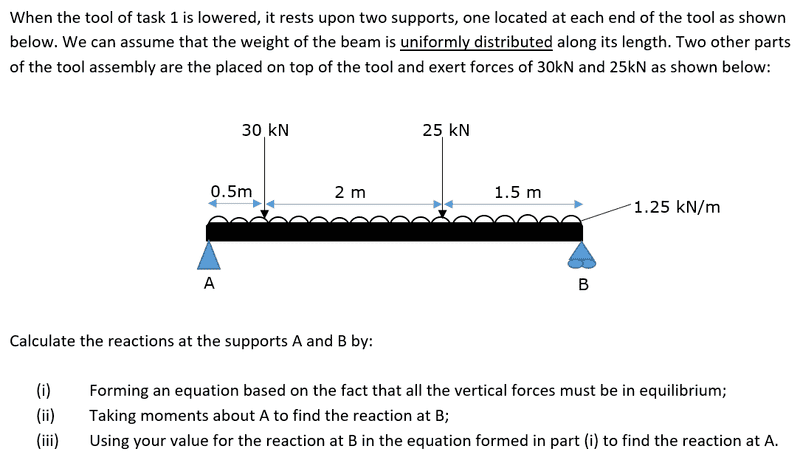# Calculating Reactions (Equations and Moments)

## Homework Statement

Need help at "Forming an equation based on the fact that all the vertical forces must be in equilibrium". I'm to calculate the reactions at the supports A and B by doing:

(i) Forming an equation based on the fact that all the vertical forces must be in equilibrium
(ii) Taking moments about A to find the reaction at B
(iii) Using your value for the reaction at B in the equation formed in part (i) to find the reaction at A## Homework Equations

I thought that the equation that I need to form would have been an equation for finding moments,
moment = force x distance.

## The Attempt at a Solution

For part (i) I done:
M = (-30 x 0.5) + (-25 x 2.5)
= -77.5

It states that they are in equilibrium so does that mean I need to add '(30 x 0.5) + (25 x 2.5)' to the equation so that it become an equilibrium? Or is what I'm doing for part (i) actually what I should be doing for part (ii) as I need to take moments for that section of the question? If so then what equation must I form for part (i)?

Part (i), I believe, is simply summing all of the vertical forces and setting equal to 0. In other words, the sum of forces up is equal to the sum of forces down.

Part (ii), I believe, involves taking the sum of moments about point A (one of those moments being the upward force at point B) and setting them equal to 0. Or, as I prefer to think about it, the sum of CW torques is equal to the sum of CCW torques.

What I didn't understand is what that 1.25 kN/m is referring to. Also, I didn't see a weight for the tool that is laying on the two supports.

And yes, moment = force x distance.

Hmmm. It's hard not to just give the answer. Ok, you say you expected to be thinking about moments. That is one thing to think about, and you know the rotational form of Newton's law

Sum of torques = rotational Inertia x angular acceleration

Since the beam isn't spinning. Sum of torques = 0 about any axis you care to pick. That may come in handy at some point.

However, the beam also isn't moving, isn't accelerating. Do you know some similar facts for linear motion?